# Pytorch中的四种经典Loss源码解析

2020-12-30 21:12| 发布者: 炼数成金_小数| 查看: 103177| 评论: 0|原作者: zzk|来自: GiantPandaCV

 笔者最近在OneFlow框架对齐实现Pytorch相关Loss代码，其中也涉及到部分源码解读，数学特殊操作等知识，于是想写篇文章简单总结一下。关于Pytorch的Loss源码了解过Pytorch的应该知道其历史包袱比较重，它吸收了Caffe2的底层代码，然后自己借用这部分底层代码来写各种OP的逻辑，最后再暴露出一层Python接口供用户使用。因此第一次接触Pytorch源代码可能有点不太熟悉，基本上Pytorch大部分OP逻辑实现代码都放在 Aten/native下，我们这里主要是根据Loss.cpp来进行讲解MarginRankingLossRankingLoss系列是来计算输入样本的距离，而不像MSELoss这种直接进行回归。其主要思想就是分为 Margin 和 Ranking。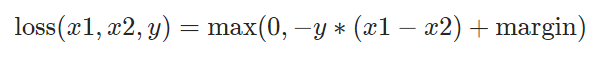MarginRankingLoss公式Margin 这个词是页边空白的意思，平常我们打印的时候，文本内容外面的空白就叫 Margin。而在Loss中也是表达类似的意思，相当于是一个固定的范围，当样本距离（即Loss）超过范围，即表示样本差异性足够了，不需要再计算Loss。Ranking 则是排序，当target=1，则说明x1排名需要大于x2；当target=2，则说明x2排名需要大于x1。其源码逻辑也很简单，就是根据公式进行计算，最后根据reduction类型来进行 reduce_mean/sum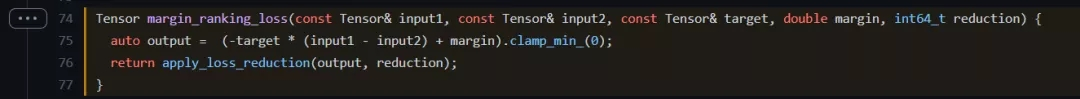Pytorch的MarginRankingLoss代码下面是对应的numpy实现代码def np_margin_ranking_loss(input1, input2, target, margin, reduction):    output = np.maximum(0, -target*(input1 - input2) + margin)    if reduction == "mean":        return np.mean(output)    elif reduction == "sum":        return np.sum(output)    else:        return outputTripletMarginLossTripletLoss最早是在 FaceNet 提出的，它是用于衡量不同人脸特征之间的距离，进而实现人脸识别和聚类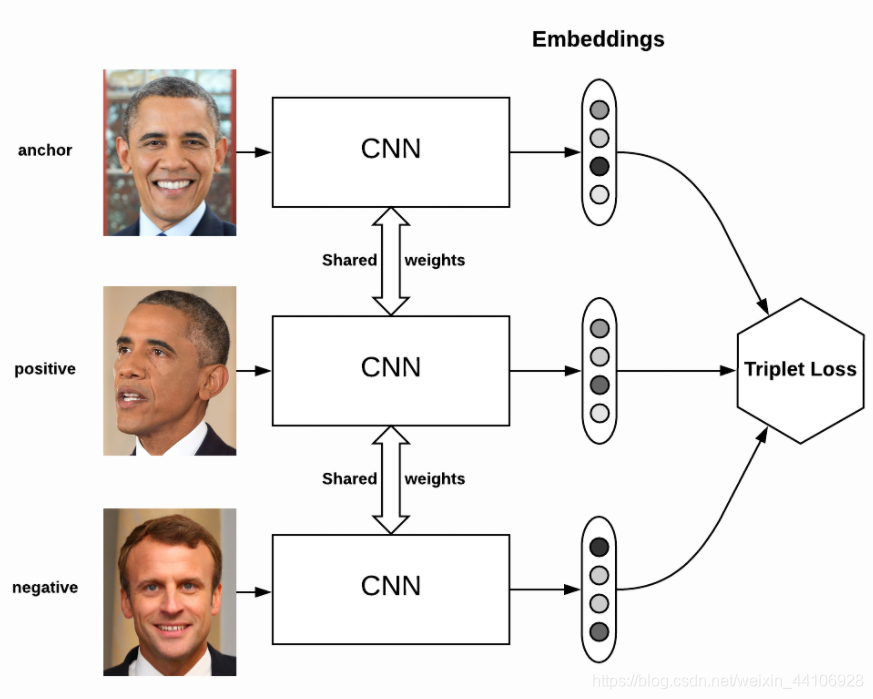TripletLoss而TripletMarginLoss则是结合了TripletLoss和MarginRankingLoss的思想，具体可参考 Learning local feature descriptors with triplets and shallow convolutional neural networks其公式如下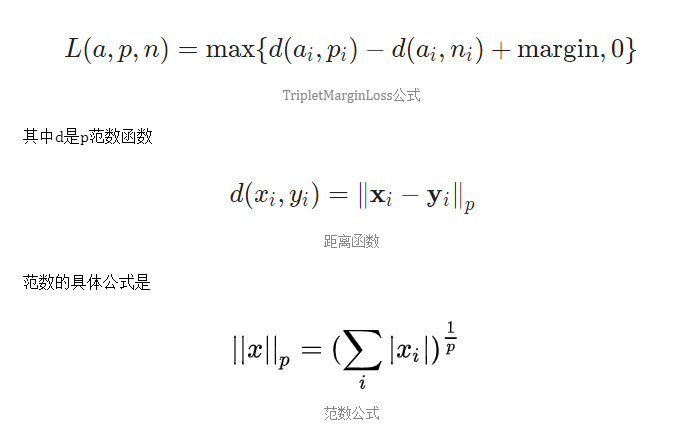该Loss针对不同样本配对，有以下三种情况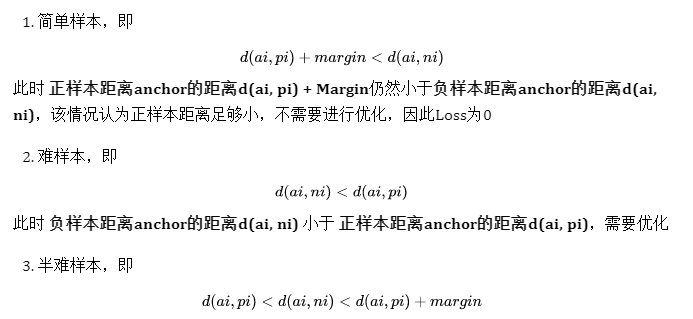此时虽然 负样本距离anchor的距离d(ai, ni) 大于 正样本距离anchor的距离d(ai, pi)，但是还不够大，没有超过 Margin，需要优化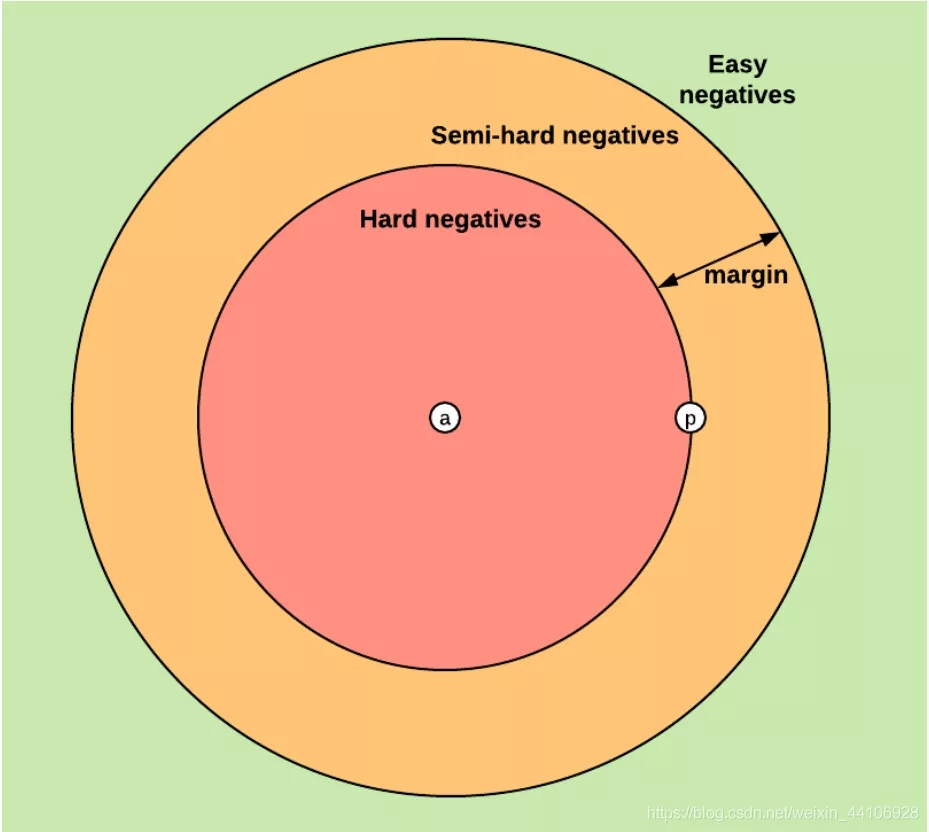此外论文作者还提出了 swap 这个概念，原因是我们公式里只考虑了anchor距离正类和负类的距离，而没有考虑正类和负类之间的距离，考虑以下情况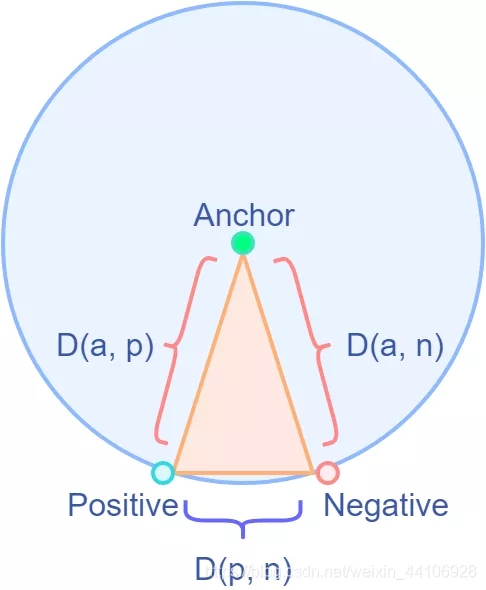可能Anchor距离正样本和负样本的距离相同，但是负样本和正样本的距离很近，不利于模型区分，因此会做一个swap，即交换操作，在代码里体现的操作是取最小值。## 伪代码if swap:  D(a, n) = min(D(a,n), D(p, n))这样取了最小值后，在Loss计算公式中，Loss值会增大，进一步帮助区分负样本。有了前面的铺垫，我们理解Pytorch的TripletMarginRankingLoss源码也非常简单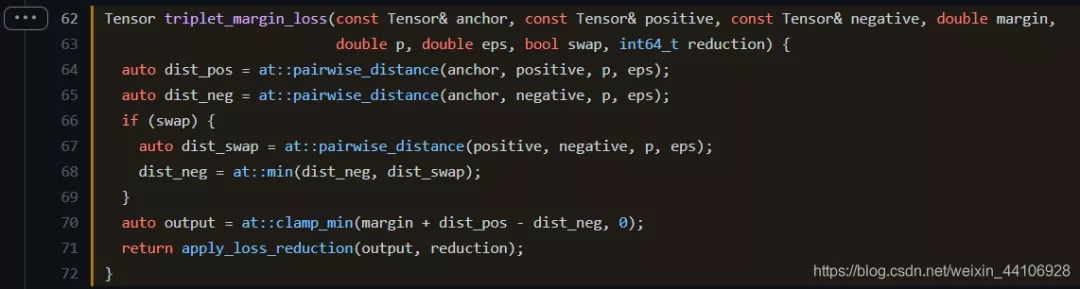TripletMarginLoss源码at::pairwise_distance是距离计算函数，首先计算出了anchor与正类和负类的距离。 然后根据参数swap，来确定是否考虑正类和负类之间的距离。最后output就是按照公式进行计算，下面是numpy的对应代码def np_triplet_margin_loss(anchor, postive, negative, margin, swap, reduction="mean", p=2, eps=1e-6):    def _np_distance(input1, input2, p, eps):     # Compute the distance (p-norm)        np_pnorm = np.power(np.abs((input1 - input2 + eps)), p)        np_pnorm = np.power(np.sum(np_pnorm, axis=-1), 1.0 / p)        return np_pnorm    dist_pos = _np_distance(anchor, postive, p, eps)    dist_neg = _np_distance(anchor, negative, p, eps)    if swap:        dist_swap = _np_distance(postive, negative, p, eps)        dist_neg = np.minimum(dist_neg, dist_swap)    output = np.maximum(margin + dist_pos - dist_neg, 0)    if reduction == "mean":        return np.mean(output)    elif reduction == "sum":        return np.sum(output)    else:        return output这里比较容易踩坑的是p范数的计算，因为当p=2，根据范数的公式，如果输入有负数是不合法的， 比如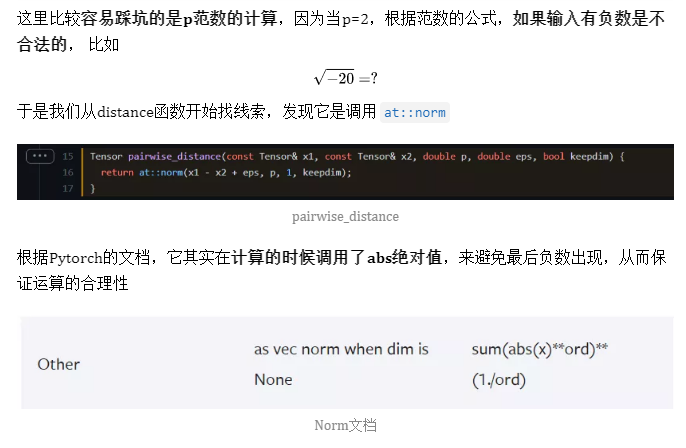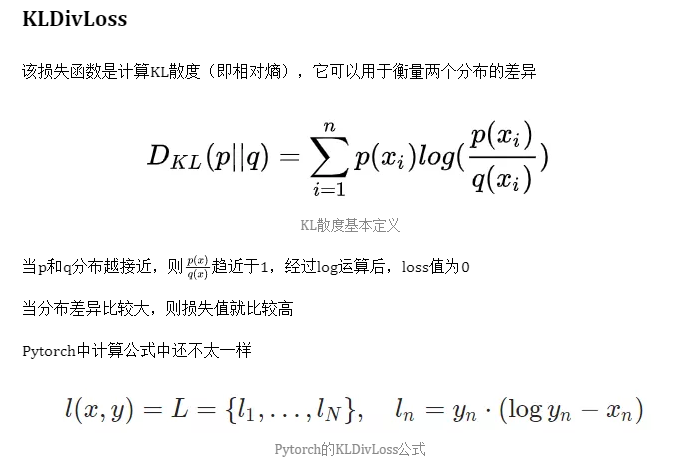下面我们看看Pytorch对应的源码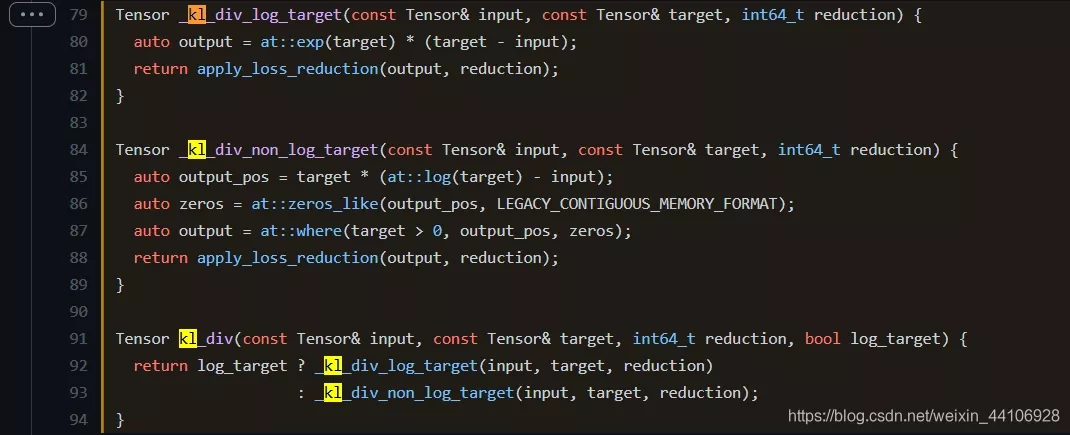KLDivLoss源码首先可以观察到，除了常规的input，target，reduction，还有一个额外的参数 log_target，用于表示target是否已经经过log运算。根 据这个参数，KLDivLoss进而分成两个函数 _kl_div_log_target 和 _kl_div_non_log_target 实现。_kl_div_log_target 的实现比较简单，就是按照公式进行计算而  _kl_div_non_log_target  有些许不同，因为target的数值范围不确定，当为负数的时候，log运算时不合法的。因此Pytorch初始化了一个全0数组，然后在最后的loss计算中，在target小于0的地方填0，避免nan数值出现下面是对应的numpy实现代码def np_kldivloss(input, target, log_target, reduction="mean"):    if log_target:        output = np.exp(target)*(target - input)    else:        output_pos = target*(np.log(target) - input)        zeros = np.zeros_like(input)        output = np.where(target>0, output_pos, zeros)    if reduction == "mean":        return np.mean(output)    elif reduction == "sum":        return np.sum(output)    else:        return outputBCEWithLogitsLoss熟悉二分类交叉熵损失函数BCELoss的应该知道，该函数输入的是个分类概率，范围在0~1之间，最后计算交叉熵。我们先看下该损失函数的参数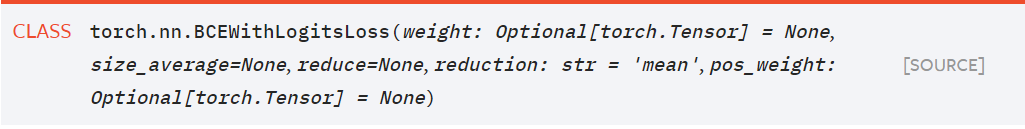BCEWithLogitsLoss参数weight 表示最后loss缩放权重reduction 表示 最后是做mean, sum, none 操作pos_weight 表示针对正样本的权重，即positive weight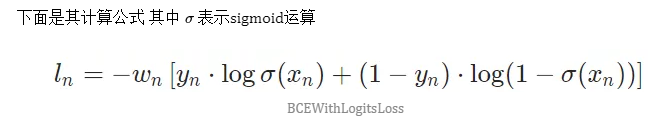BCEWithLogitsLossBCEWithLogitsLoss 相当于 sigmoid + BCELoss，但实际上 Pytorch为了更好的数值稳定性，并不是这么做的，下面我们看看对应的源代码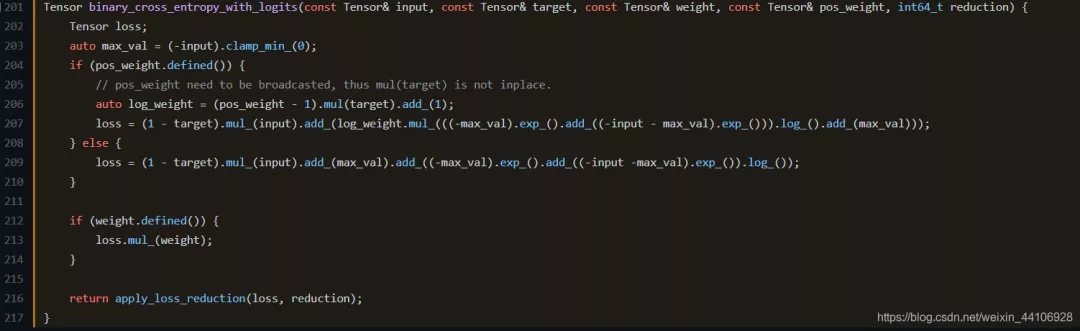Pytorch的BCEWithLogitsLoss源码这段源代码其实看的不太直观，我们可以看下numpy对应的代码def np_bce_with_logits_loss(np_input, np_target, np_weight, np_pos_weight, reduction="mean"):    max_val = np.maximum(-np_input, 0)    if np_pos_weight.any():        log_weight = ((np_pos_weight - 1) * np_target) + 1        loss = (1 - np_target) * np_input        loss_1 = np.log(np.exp(-max_val) + np.exp(-np_input - max_val)) + max_val        loss += log_weight * loss_1    else:        loss = (1 - np_target) * np_input        loss += max_val        loss += np.log(np.exp(-max_val) + np.exp(-np_input - max_val))    output = loss * np_weight    if reduction == "mean":        return np.mean(output)    elif reduction == "sum":        return np.sum(output)    else:        return output因为涉及到了sigmoid运算，所以有以下公式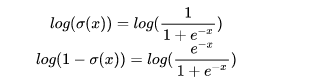计算中，如果x过大或过小，会导致指数运算出现上溢或下溢，因此我们可以 用 log-sum-exp 的技巧来避免数值溢出，具体可以看下面公式推导（特此感谢德澎！）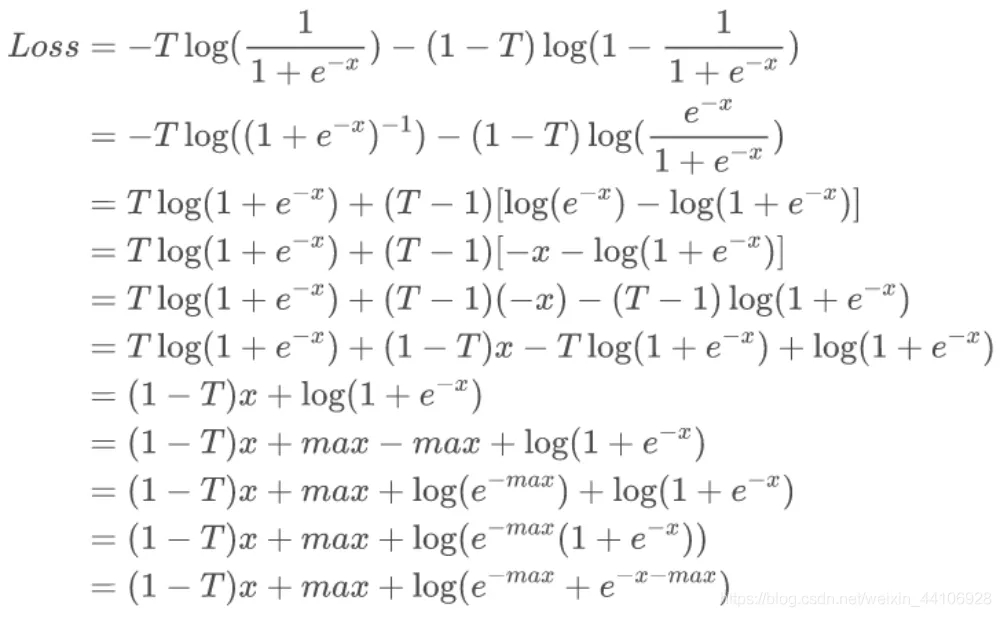公式推导总结看源代码没有想象中那么难，只要破除迷信，敢于尝试，你也能揭开源码的神秘面纱~相关链接triplet-loss(https://omoindrot.github.io/triplet-loss)FaceNet(https://arxiv.org/pdf/1503.03832.pdf)TripletMarginLoss(http://www.bmva.org/bmvc/2016/papers/paper119/paper119.pdf)RankingLoss(https://gombru.github.io/2019/04/03/ranking_loss/)声明：文章收集于网络，版权归原作者所有，为传播信息而发，如有侵权，请联系小编删除，谢谢！欢迎加入本站公开兴趣群商业智能与数据分析群兴趣范围包括：各种让数据产生价值的办法，实际应用案例分享与讨论，分析工具，ETL工具，数据仓库，数据挖掘工具，报表系统等全方位知识QQ群：81035754

## 热门频道

• 大数据
• 商业智能
• 量化投资
• 科学探索
• 创业GMT+8, 2021-6-25 19:00 , Processed in 0.128963 second(s), 24 queries .